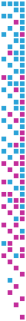## Information

• Publication Type: Master Thesis
• Workgroup(s)/Project(s):
• Date: November 2017
• Diploma Examination: 14. November 2017
• First Supervisor: Michael Wimmer
• Keywords: error metric, global illumination

## Abstract

This work aims at improving methods for measuring the error of unbiased, physically based light-transport algorithms. State-of-the-art papers show algorithmic improvements via error measures like Mean Square Error (MSE) or visual comparison of equal-time renderings. These methods are unreliable since outliers can cause MSE variance and visual comparison is inherently subjective. We introduce a simple proxy algorithm: pure algorithms produce one image corresponding to the computation budget N. The proxy, on the other hand, averages N independent images with a computation budget of 1. The proxy algorithm fulfils the preconditions for the Central Limit Theorem (CLT), and hence, we know that its convergence rate is (1/N). Since this same convergence rate applies for all methods executed using the proxy algorithm, comparisons using variance- or standard-deviation-per-pixel images are possible. These per-pixel error images can be routinely computed and allow comparing the render quality of different lighting effects. Additionally, the average of pixel variances is more robust against outliers compared to the traditional MSE or comparable metrics computed for the pure algorithm. We further propose the Error Spectrum Ensemble (ESE) as a new tool for evaluating lighttransport algorithms. It summarizes expected error and outliers over spatial frequencies. ESE is generated using the data from the proxy algorithm: N error images are computed using a reference, transformed into Fourier power spectra and compressed using radial averages. The descriptor is a summary of those radial averages. In the results, we show that standard-deviation images, short equal-time renderings, ESE and expected MSE are valuable tools for assessing light-transport algorithms.

No further information available.

## BibTeX

```@mastersthesis{CELAREK-2017-QCL,
title =      "Quantifying the Convergence of Light-Transport Algorithms",
year =       "2017",
abstract =   "This work aims at improving methods for measuring the error
of unbiased, physically based light-transport algorithms.
State-of-the-art papers show algorithmic improvements via
error measures like Mean Square Error (MSE) or visual
comparison of equal-time renderings. These methods are
unreliable since outliers can cause MSE variance and visual
comparison is inherently subjective. We introduce a simple
proxy algorithm: pure algorithms produce one image
corresponding to the computation budget N. The proxy, on the
other hand, averages N independent images with a computation
budget of 1. The proxy algorithm fulfils the preconditions
for the Central Limit Theorem (CLT), and hence, we know that
its convergence rate is (1/N). Since this same convergence
rate applies for all methods executed using the proxy
algorithm, comparisons using variance- or
standard-deviation-per-pixel images are possible. These
per-pixel error images can be routinely computed and allow
comparing the render quality of different lighting effects.
Additionally, the average of pixel variances is more robust
against outliers compared to the traditional MSE or
comparable metrics computed for the pure algorithm. We
further propose the Error Spectrum Ensemble (ESE) as a new
tool for evaluating lighttransport algorithms. It summarizes
expected error and outliers over spatial frequencies. ESE is
generated using the data from the proxy algorithm: N error
images are computed using a reference, transformed into
Fourier power spectra and compressed using radial averages.
The descriptor is a summary of those radial averages. In the
results, we show that standard-deviation images, short
equal-time renderings, ESE and expected MSE are valuable
tools for assessing light-transport algorithms.",
month =      nov,
address =    "Favoritenstrasse 9-11/E193-02, A-1040 Vienna, Austria",
school =     "Institute of Computer Graphics and Algorithms, Vienna
University of Technology ",
keywords =   "error metric, global illumination",
URL =        "https://www.cg.tuwien.ac.at/research/publications/2017/CELAREK-2017-QCL/",
}
```